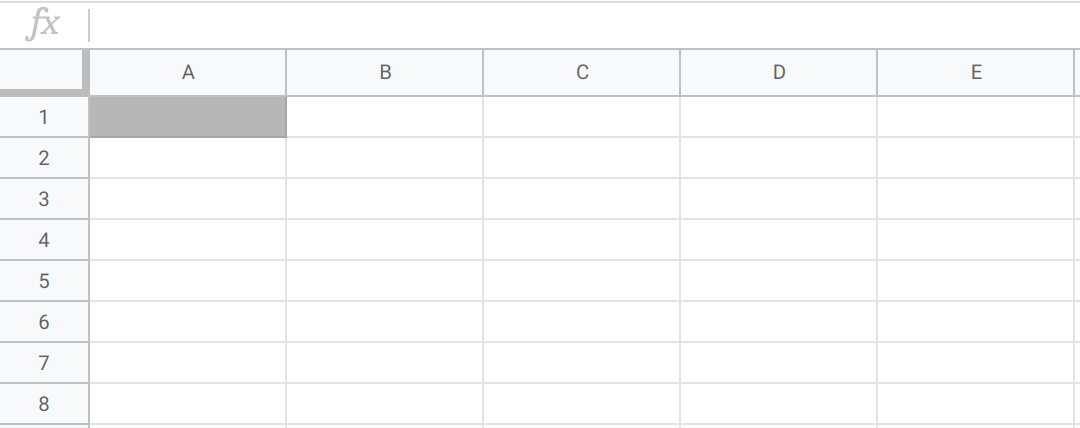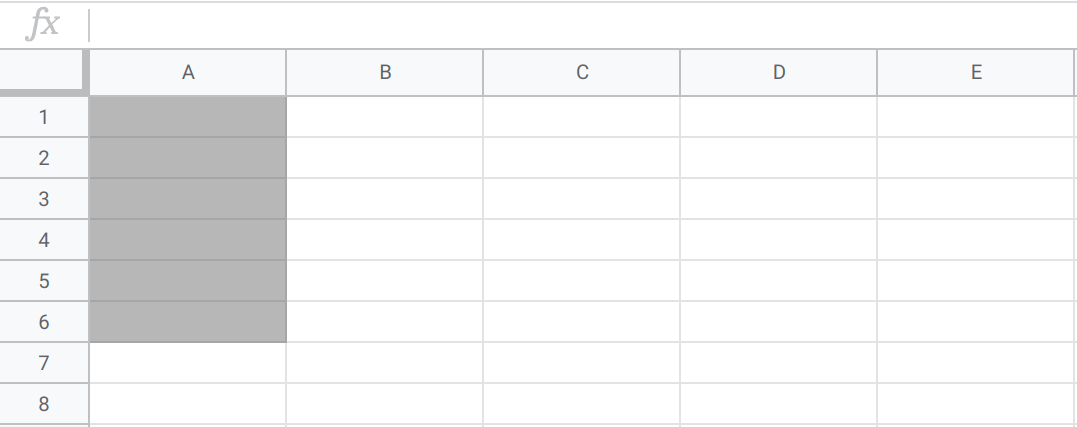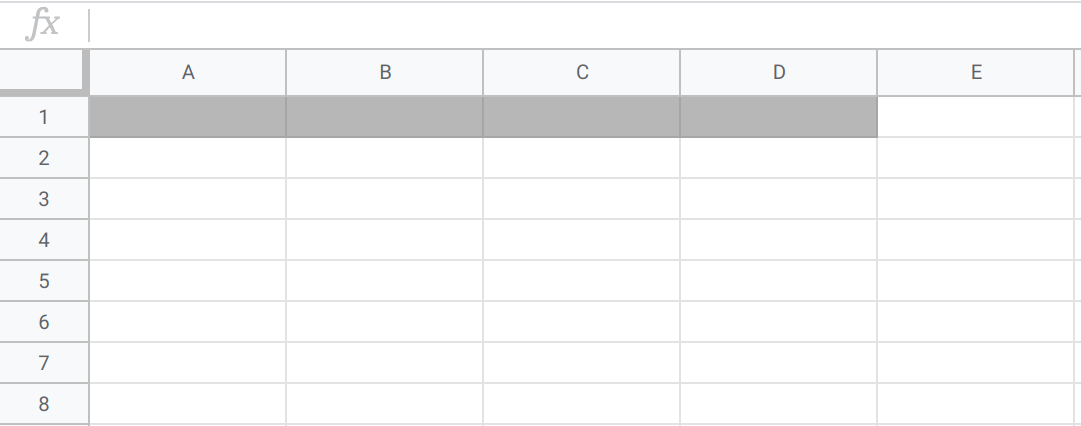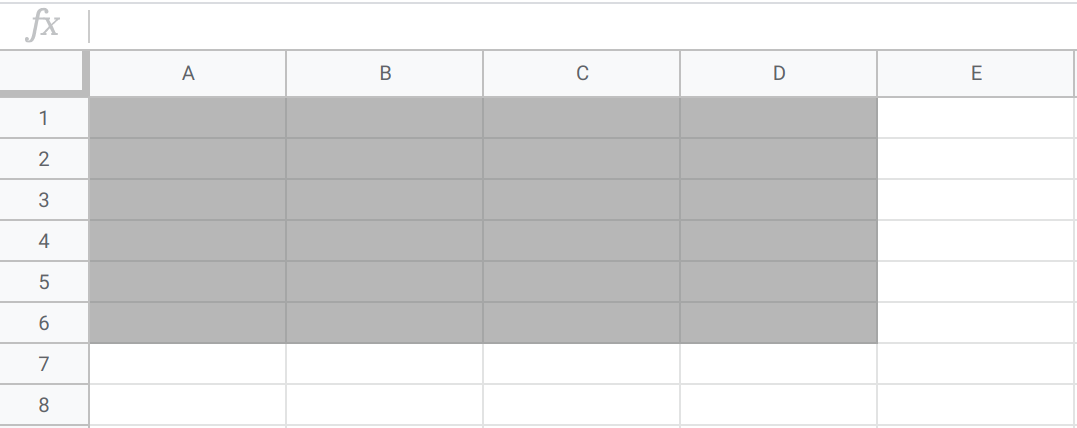# Count and Sum¶

Two of the most basic but most used statistics are the count and sum of a dataset.

Count Definitions

The count of a dataset is the number of observations in the dataset. This applies to all variables.

Sum Definition

The sum of a dataset is the addition of all values in the dataset. This applies only to quantitative variables.

## Applications and Examples¶

The reason that the count is so important is that it can be an indication of how useful the dataset is. For example, suppose you’re trying to find the average height of students at your college of 800 people, so you conduct a survey collecting students’ heights. If your survey contains only 2 heights, then using these results to calculate an average won’t be very useful, since this summary statistic is only representative of 2 students. If, however, your survey contains 600 heights, calculating an average from these results will be more reliable, since this statistic represents 600 of the 800 students.

As an example of using count and sum, suppose you are throwing a homework party. You ask your friends to RSVP if they are attending and to submit how many pizza slices they would like to eat, through a survey. Knowing how many people would be attending will be helpful to you when you purchase snacks, and you can use the count to calculate the number of guests. You could also take the sum of the number of pizza slices your friends want in order to get a better idea of how many pizzas to order.

Calculating these statistics in Sheets is fairly straightforward. For these and other statistics, you’ll need to know cell range notation. To refer to a range of cells in Sheets, type the first cell in the range and the last cell in the range, separated by a colon. For example:

• “A1” refers to cell A1• “A1:A6” means all cells in the A column from rows 1 through 6• “A1:D1” means all cells in the 1 row from columns A through D• “A1:D6” means all cells in rows 1 through 6 and columns A through DCount and Sum Sheets Syntax

• The COUNT function returns the number of non-empty cells in a range. The syntax is =COUNT(cell range).

• The SUM function returns the addition of a cell range. The syntax is =SUM(cell range).

## Example: Spotify Premium¶

You and your roommates have been wanting to get Spotify Premium on a family plan for quite a while. There are five of you, and you know that the plan allows up to six accounts for $15 per month. However, you are all on a very tight college-student budget, and have very little cash to spare. You have each gone through your expenses and have narrowed down exactly how much money you each have to spare per month. Now you want to know, if you pool your money together, whether you will have enough for Spotify Premium! You could use a calculator, but you prefer storing the data in a sheet, because if any values were to change in the future, you could easily update the sheet. First, you input all of your spare money into the sheet. (Note you can click the “$” symbol to format the numbers as currency.)

First, use the COUNT function to verify that there are five rows in this dataset, one for each person on the plan.

Now, use the SUM function to add up all the values.

Alas, you and your roommates are agonizingly short of the required \$15 per month! You’ll have to keep trying to save just 90 cents more per month to have enough for Spotify Premium.

You have attempted of activities on this page